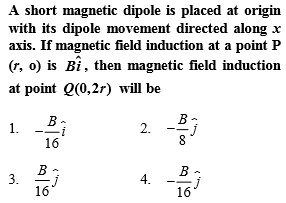NEET Questions Solved(1)

$\mathrm{B}=\frac{{\mathrm{\mu }}_{0}}{4\mathrm{\pi }}\frac{2\mathrm{M}}{{\mathrm{r}}^{3}}\phantom{\rule{0ex}{0ex}}\mathrm{B}\text{'}=\frac{{\mathrm{\mu }}_{0}}{4\mathrm{\pi }}\frac{\mathrm{M}}{8{\mathrm{r}}^{3}}\phantom{\rule{0ex}{0ex}}\mathrm{B}\text{'}=\frac{\mathrm{B}}{16}$

Difficulty Level:

• 21%
• 32%
• 23%
• 26%